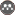Volume 26, Issue 3 (Fall 2022)                   jwss 2022, 26(3): 91-107 | Back to browse issues page

BibTeX | RIS | EndNote | Medlars | ProCite | Reference Manager | RefWorks
Send citation to:Shahinejad B, Parsaei A, Haghizadeh A, Arshia A, Shamsi Z. Modeling and Estimating the Dimensions of Stable Alluvial Channels using Soft Calculations. jwss 2022; 26 (3) :91-107
URL: http://jstnar.iut.ac.ir/article-1-4199-en.html
Lorestan University , babak_shd1355@yahoo.com
Abstract:   (939 Views)
In this research, soft computational models including multiple adaptive spline regression model (MARS) and data group classification model (GMDH) were used to estimate the geometric dimensions of stable alluvial channels including channel surface width (w), flow depth (h), and longitudinal slope (S) and the results of the developed models were compared with the multilayer neural network (MLP) model. To develop the models, the flow rate parameters (Q), the average particle size in the floor and body (d50) as well as the shear stress (t) as input and the parameters of water surface width (w), flow depth (h), and longitudinal slope (S) were used as output parameters. Soft computing models were developed in two scenarios based on raw parameters and dimensionless form independent and dependent parameters. The results showed that the statistical characteristics in estimating w, the best performance is related to the MARS model, whose statistical indicators of accuracy in the training stage are R2 = 0.902, RMSE=1.666 and in the test phase is R2 = 0.844, RMSE=2.317. In estimating the channel depth, the performance of both GMDH and MARS models is approximately equal, both of which were developed based on the dimensionless form of flow rate as the input variable. The statistical indicators of both models in the training stage are R2 » 0.90, RMSE » 8.15 and in the test phase is R2 » 0.90, RMSE = 7.40. The best performance of the developed models in estimating the longitudinal slope of the channel was related to both MARS and GMDH models, although, in part, the accuracy of the GMDH model with statistical indicators R2 = 0.942, RMSE = 0.0011 in the training phase and R2 = 0.925, RMSE = 0.0014 in the experimental stage is more than the MARS model.Rights and permissionsThis work is licensed under a Creative Commons Attribution-NonCommercial 4.0 International License.